# Prediction with Classification in Azure Machine Learning## Introduction

After discussing Regression in the previous article, let us discuss the techniques for Classification in Azure Machine learning in this article. Like regression, classification is also the common prediction technique that is being used in many organizations. Before the regression we have discussed basic cleaning techniques, feature selection techniques and Principal component analysis in previous articles, now we will be looking at data classification techniques in azure

## Different Types of Classification Techniques

The Classification technique is used to classify and can be used to determine risk and non-risk customers for a
banking sector, detect spam or non-spam emails, identifying whether the prospective customer will buy a bike or not,
classify plants and animals.

There are different classification techniques available in Azure Machine Learning to support various features as
shown in the following screen.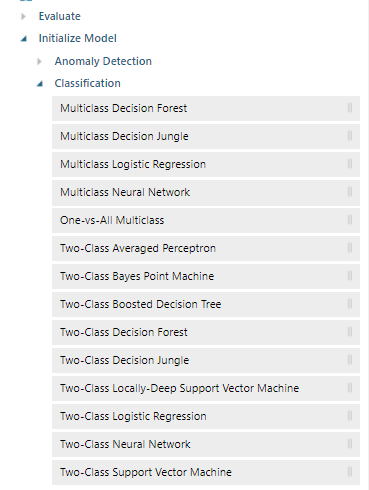As you see in the above screenshot, there are so many classifications options in Azure Machine Learning and from
which you can use for different purposes. Therefore, it is essential to choose the correct Classification that suits

Mainly classification algorithms have two types of algorithms, Two-class and Multi-Class. Two class algorithms are
much suited for data that has two classes. For example, a bike buyer has two classes such as bike buyers or not. The
multi-class algorithm is used to classify data set with multiple classes. For example, if you want to classify
animals, you need to use multi-class classification algorithms.

Let us see the basic properties and usage of techniques of classification in Azure Machine Learning in the following
table:

 Algorithm Properties Multiclass Logistic Regression Fast training Time, Linear Model Multiclass Neural Network Higher accuracy, larger training times Multiclass Decision Forest Higher accuracy, faster training times Multiclass Boosted Decision Tree Scalable faster training time. Two-class Support vector machine A Linear model for data set with less than 100 features Two-class averaged perception Linear and faster training model. Two-class decision forest Higher accuracy Two-class Logistic Regression Linear and faster training model. Two-class Boosted Decision Tree Higher accuracy but more resources are consumed. Two-class Neural Network Higher accuracy but more training time is required.

Let us see how to create an experiment with the utilization of Classification technique in Azure Machine Learning.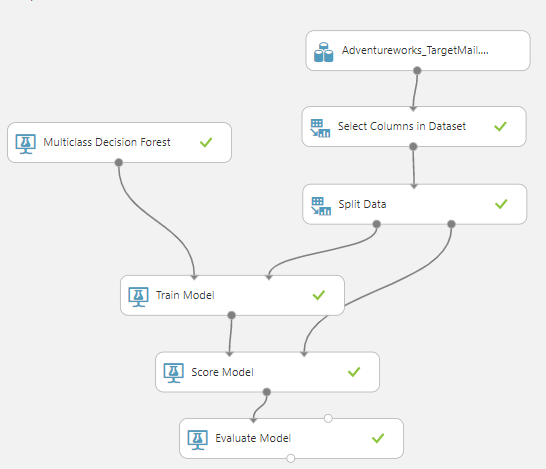Though we discussed how to create an experiment in Azure Machine Learning, let us quickly walk through how to create an experiment.

First of all, let us use the sample AdventureWorks data set. Since we don’t need all columns, such as an address,
name, etc., we will select only the columns that we think will contribute to the bike buyer. To select the necessary
columns, we will use Select Columns in Dataset Control. In the case of prediction, we need a
dataset to be divided into train and test that is done from Split Control. In this, we have divided the dataset with a 70/30 rule. Then the train data set is linked to the train model control and in the train model control, we have used the BikeBuyer column as the prediction column. The train model is fed with the different classification models which will be discussed later in the article. After the model is trained Score model is used to test the model by feeding the test dataset to the Score Model. Finally, the
evaluation is done from the Evaluate Model control.

The output of the Evaluate model is differs depending on whether the algorithm is a multi-class or two-class. The
Multi-class classification can have multiple classifications such as multiple animals, multiple plant types etc. In
the two-class classification, there will be only two classes of classification such as whether the bike buyer is
not, whether the mail is spam or not etc.

Let us first look at the Evaluation in the Multiclass for the Classification in Azure Machine Learning.

In the above screenshot, you can see that there are three additional columns that are included in the output for classification in Azure Machine Learning. These additional columns indicated what are the probabilities for each class. Scored labels will be the class that has the highest probability.

The following table shows different evaluation parameters for different multi-class algorithms in classification.

 Accuracy Precision Recall Multiclass Decision Forest 0.80 0.80 0.80 Multiclass Decision Jungle 0.75 0.75 0.75 Multiclass Logistic Regression 0.65 0.65 0.65 Multiclass Neural Network 0.75 0.75 0.75

Let us look at the evaluation of two-class classification which is different from the multiclass classification technique in Azure Machine Learning.

There are three charts to evaluate the two-class classification in Azure Machine Learning. One of them is the ROC
curve. ROC or Receiver Operation Curve is a visual tool to find
the accuracy of the model. Ideally, the ROC curve should be over the random as shown in the below screenshot.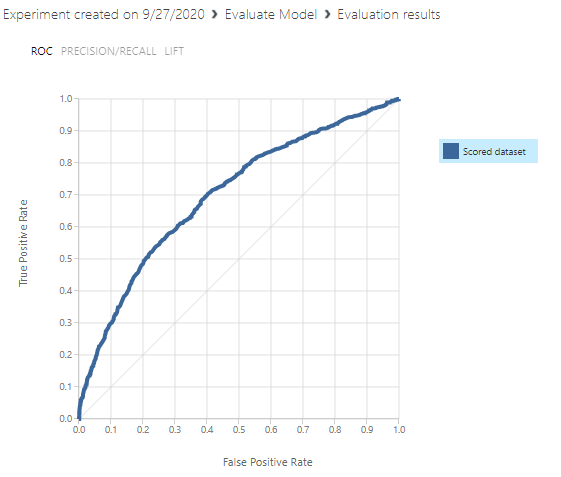As you see in the above screen, the ROC curve is higher than the standard normal curve.

The next important graph is the PRECISION and RECALL graph.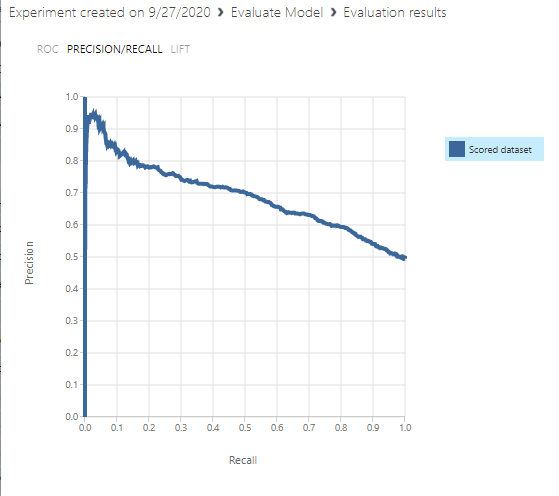Precision is out of the predicted positive the data set how much is actually positive and recall is out of actually positive, how much is predicted as positive. Depending on the case, you might be looking at better precision or recall.

The Lift chart is another chart that is shown in the next screenshot.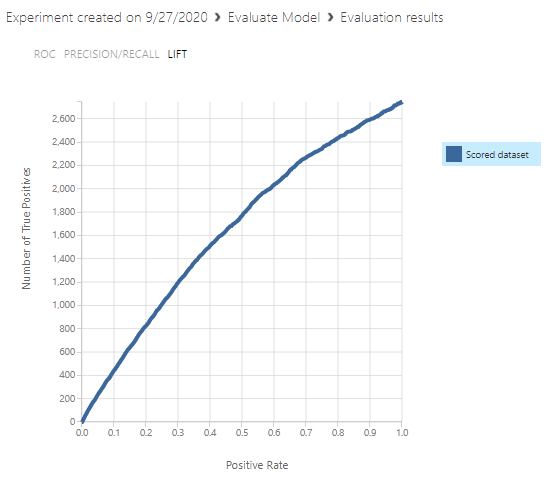The confusion matrix is an important matrix to evaluate classification in Azure Machine learning.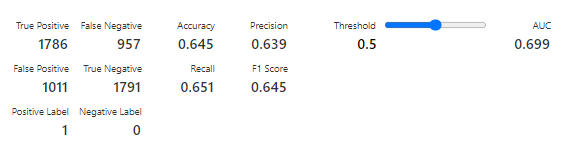F1 score which is the harmonic mean of precision and recall is another important measure that can be retrieved in the techniques of Classification in Azure Machine Learning. However, the most correct accuracy measure in two-classification techniques is Matthews correlation coefficient or MCC. This is not available in Azure Machine
Learning.

Let us compare the evaluation parameters for different classification techniques.

 Techniques Accuracy Precision Recall F1 Measure Two-Class Averaged Perceptron 0.645 0.639 0.651 0.645 Two-Class Bayes Point Machine 0.650 0.640 0.668 0.653 Two-Class Boosted Decision Tree 0.800 0.788 0.816 0.801 Two-Class Decision Forest 0.797 0.793 0.799 0.796 Two-Class Decision Jungle 0.754 0.748 0.758 0.753 Two-Class Locally-Deep Support Vector Machine 0.735 0.729 0.737 0.733 Two-Class Logistic Regression 0.650 0.641 0.667 0.653 Two-Class Neural Network 0.756 0.767 0.728 0.747 Two-Class Support Vector Machine 0.641 0.640 0.623 0.632

By observing the above table, it can be seen that Two-Class Boosted Decision Tree has better accuracy for this data
set. However, Two-Class Decision Forest has marginally higher precision than Two-Class Decision Tree. This shows
that depending on the need and the data set, you need to choose the correct techniques o classification in Azure
Machine Learning. Further, there are different parameters for each technique that has to be configured in order to
define a better model for prediction using classification techniques.

After the model is created, the next task is to perform prediction as we did in the previous articles. For this, we
need to deploy the model as a web service from the Azure Machine Learning.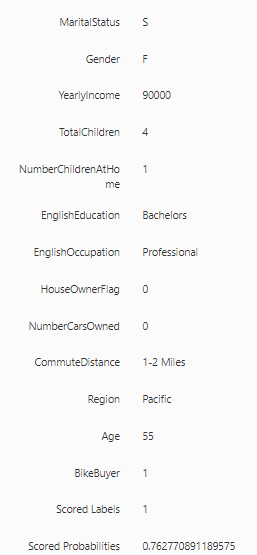Once you provide data, web service will predict whether the prospective customer is a bike buyer or not. In the
above example, the customer is a bike buyer with a 77% probability.

## Conclusion

In this article, we looked at different algorithms for Classification in Azure Machine Learning. In azure machine
learning, there are rich controls to model Classification. There are mainly two types of classification which are
multiclass and two-class. There are several evaluation techniques in classification such as Accuracy, Precision,
Recall and F1. The two-class classification has many graphs to define the accuracy of the models, such as ROC and
LIFT charts.

## Further References